Circles
edHelper subscribers - Create a new printable

Number of Keys
 Select the number of different printables: 1 key 2 keys 3 keys 4 keys 5 keys

Number of Pages (for each key)
 Select the number of pages: 1 page 2 pages 3 pages 4 pages

Include an answer key (answer keys will be at the end of the printable)
High School Geometry
High School Geometry
Math

 Name _____________________________Date ___________________ (Key 1 - Answer ID # 1004418)
1.

Draw a circle and inscribe an obtuse triangle in the circle.

2.

How are circles defined and named?

3.

What is a semicircle?

4.

What is an inscribed polygon?

5.

If a minor arc makes a central angle of 79°, what is the measure of the angle made by its complementary major arc?

6.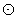P andQ are congruent circles that intersect at C and D. If the radius is 9 cm and PQ = 8 cm, what is the area of quadrilateral PCQD?

7.

An isosceles right triangle inscribed in a circle. If the length of the two equal sides is 15 cm, find the radius of the circle.

8.

What is the distance between the endpoints of a semicircle? Explain how you got your answer.

9.

What is wrong with the statement: "All radii are congruent."?

10.

Can any chord on a circle be a radius?

11.

Describe the three possible arcs that could be found on a circle.

12.

Why are three letters needed to name a major arc?

13.

Hannah is working on a sewing project. She has a circular piece of fabric, and needs to find the center. How can she do that?

14.

Eight points lie on the circumference of a circle. How many inscribed triangles can be constructed having any three of these points as vertices?

15.

The sum of all the arcs of a circle is how many degrees?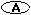90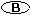360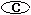180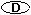720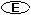27016.P andQ are congruent circles that intersect at C and D. What kind of quadrilateral must PCQD be?a squarea rhombusa trapezoida parallelogram

Circles
edHelper subscribers - Create a new printable

Number of Keys
 Select the number of different printables: 1 key 2 keys 3 keys 4 keys 5 keys

Number of Pages (for each key)
 Select the number of pages: 1 page 2 pages 3 pages 4 pages